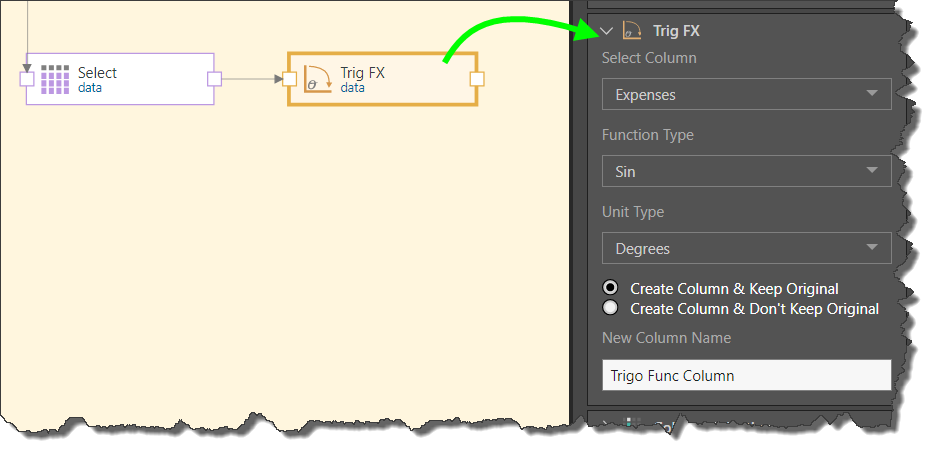# Trig FX

The Trig FX node is used to generate a new column based in a trigonometric function applied to a numeric column. This function supports the following numeric column types: Integer, Float 32, Float 64, and BigInteger.

## Configure Trig FX

Connect the Trig FX node to the Select tables of the relevant table. Go to the Properties panel and set the trigonometric preferences:

Select Column: choose the column(s) to which you want to apply the trigonometric function.

Function Type: set the trigonometric function to apply to the column.

Unit Type: choose degrees or radians.

Column Output: determine whether or not to keep the original column.

New Column Name: name the new column.### Function Type

The Trig FX node enables the following trigonometric functions:

• Sin: returns the sine of a number.
• Cos: returns the cosine of a number.
• Tan: returns the tangent of a number.
• CoTan: returns the cotangent of a number.
• ArcSin: returns the arc sine of a number.
• ArcCos: returns the arc cosine of a number.
• ArcTan: returns the arc tangent of a number.